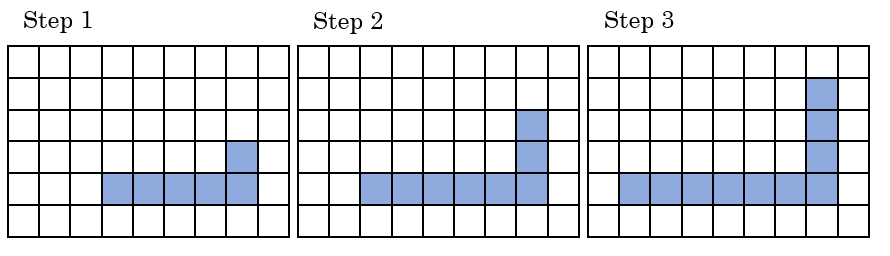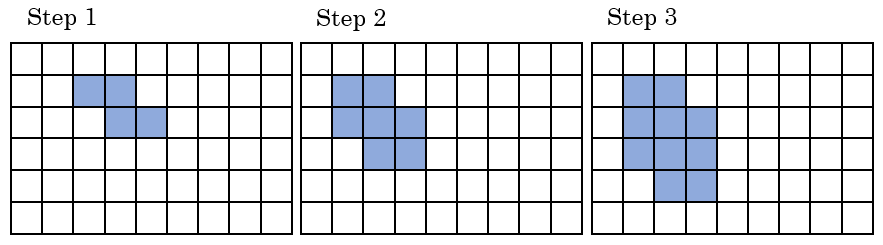# Linear Representations

In this section, you will make connections between the linear trends found in the patterns, tables, graphs, and equations.  Be sure to complete all four representations.

## Patterns

Below is the description of growth and the equation for each pattern.Pattern 1 Grows by 2, starts with 4 squares (Step 0) Equation 1 $$s=2n+4$$Pattern 2 Grows by 2, starts with 1 square Equation 2 $$s=2n+1$$Pattern 3 Grows by 3, starts with 2 squares Equation 3 $$s=3n+2$$Pattern 4 Grows by 3, starts with 1 square Equation 4 $$s=3n+1$$